## Excel Formula: Find most frequent text with criteria

In some cases, you may want to find the text which appears most frequently based on a criterion in Excel. This tutorial introduces an array formula to handle this job and gives the explanation about the arguments of this formula.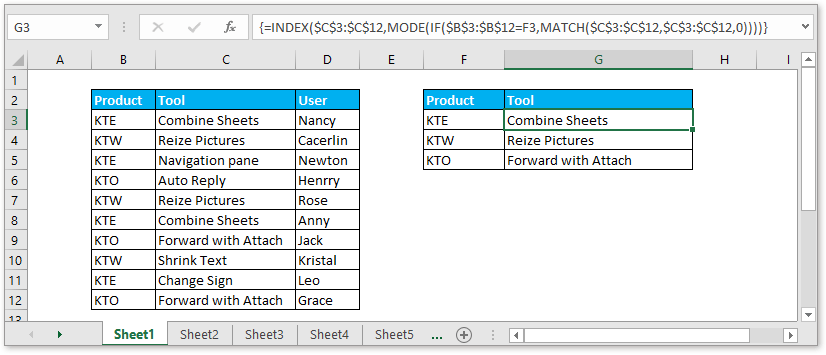Generic formula:

 =INDEX(rng_1,MODE(IF(rng_2=criteria,MATCH(rng_1,rng_1,0))))

Arguments

 Rng_1: the range of cells that you want to find the most frequent text. Rng_2: the range of cells that contain the criteria you want to use. Criteria: the condition you want to find text based on.

Return value

This formula returns the most frequent text with a specific criterion.

How this formula work

Example: There is a range of cells listing products, tools and users, now you want to find the most frequent used tool of each product. Please use below formula in Cell G3:

 =INDEX(\$C\$3:\$C\$12,MODE(IF(\$B\$3:\$B\$12=F3,MATCH(\$C\$3:\$C\$12,\$C\$3:\$C\$12,0))))

Press Shift + Ctrl + Enter keys together to get the correct result. Then drag fill handle down to apply this formula.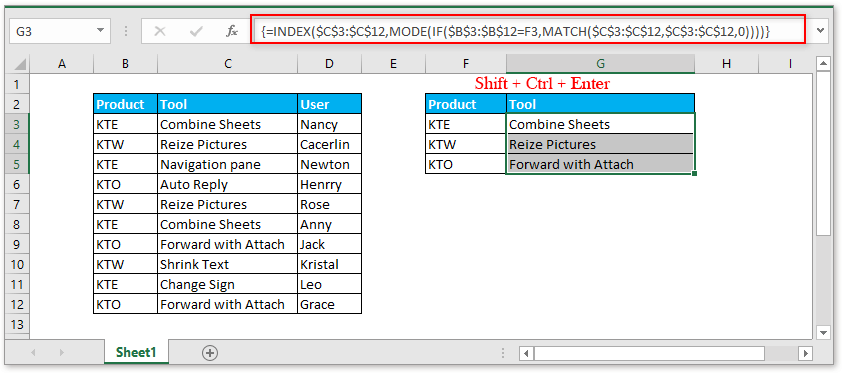Explanation

MATCH(\$C\$3:\$C\$12,\$C\$3:\$C\$12,0): the MATCH function returns the location of the lookup_value in a row or column. Here the formula returns the array result {1;2;3;4;2;1;7;8;9;7}, which finds the position of each text in range \$C\$3:\$C\$12.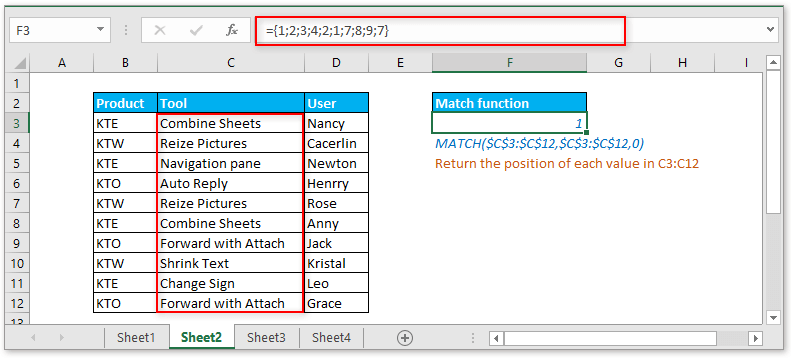IF(\$B\$3:\$B\$12=F3,MATCH(\$C\$3:\$C\$12,\$C\$3:\$C\$12,0)): the IF function is used to set a condition. Here this formula is seen as IF(\$B\$3:\$B\$12=”KTE”,{1;2;3;4;2;1;7;8;9;7}) , and the array result returns ={1;FALSE;3;FALSE;FALSE;1;FALSE;FALSE;9;FALSE}.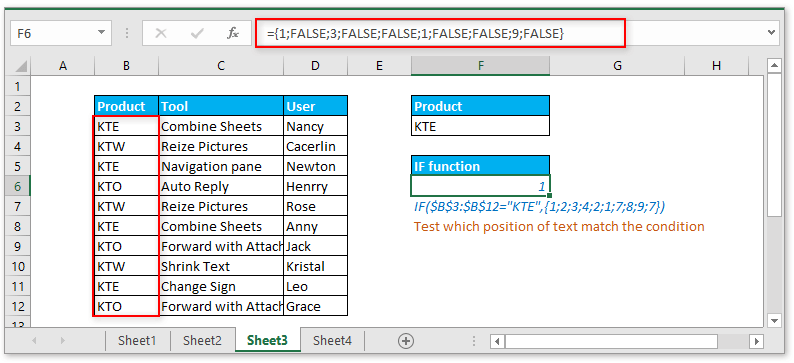MODE(IF(\$B\$3:\$B\$12=F3,MATCH(\$C\$3:\$C\$12,\$C\$3:\$C\$12,0))): MODE function finds the most frequent text in a range. Here this formula will find the most frequent number in the array result of the IF function, which can be seen as MODE({1;FALSE;3;FALSE;FALSE;1;FALSE;FALSE;9;FALSE}) and returns 1.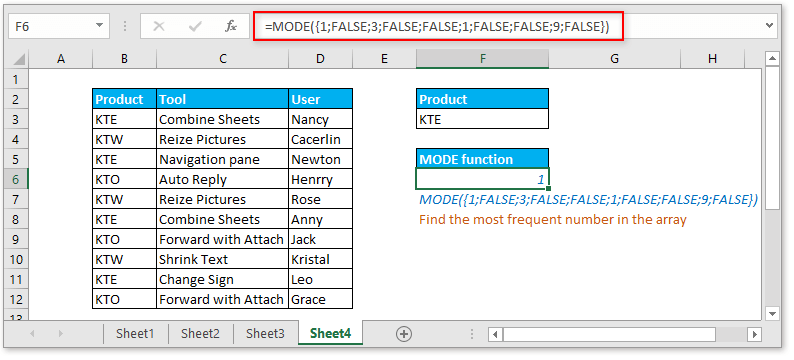INDEX function: the INDEX function returns the value in a table or array based on the given location. Here the formula. INDEX(\$C\$3:\$C\$12,MODE(IF(\$B\$3:\$B\$12=F3,MATCH(\$C\$3:\$C\$12,\$C\$3:\$C\$12,0)))) will be shrunk to INDEX(\$C\$3:\$C\$12,1).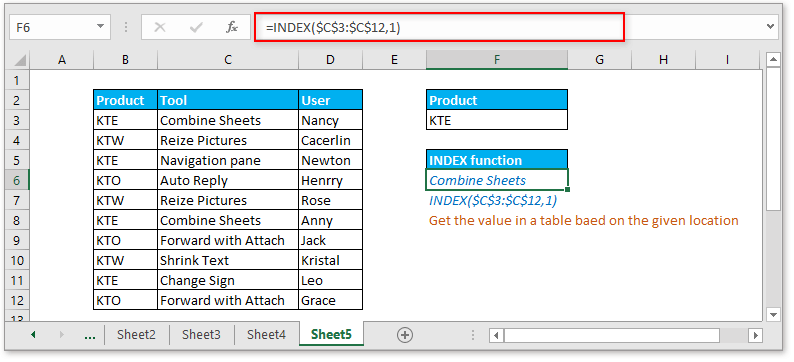Remark

If there are two or more most frequent texts, the formula will return the value which appear at first.

### Sample File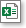Click to download sample file

#### Relative Formulas

• Check if a cell contains a specific text
To check if a cell contains some texts in range A but does not contain the texts in range B, you can use an array formula which combines the COUNT, SEARCH and AND function in Excel
• Check if a cell contains one of several values but exclude other values
This tutorial will provide a formula to quickly handle the task that check if a cell contains one of things but excluding other values in Excel and explain the arguments of the formula.
• Check if cell contains one of things
Supposing in Excel, there is a list of values in column E, you want to check if the cells in column B contain all of the values in column E, and return TRUE or FALSE.
• Check if cell contains number
Sometimes, you may want to check if a cell contains numeric characters. This tutorial provides a formula which will return TRUE if the cell contains number, FALSE if cell does not contain number.

### The Best Office Productivity Tools

#### Kutools for Excel - Helps You To Stand Out From Crowd

 Popular Features: Find, Highlight or Identify Duplicates  |  Delete Blank Rows  |  Combine Columns or Cells without Losing Data  |  Round without Formula ... Super VLookup: Multiple Criteria  |  Multiple Value  |  Across Multi-Sheets  |  Fuzzy Lookup... Adv. Drop-down List: Easy Drop Down List  |  Dependent Drop Down List  |  Multi-select Drop Down List... Column Manager: Add a Specific Number of Columns  |  Move Columns  |  Toggle Visibility Status of Hidden Columns  |  Compare Columns to Select Same & Different Cells ... Featured Features: Grid Focus  |  Design View  |  Big Formula Bar  |  Workbook & Sheet Manager | Resource Library (Auto Text)  |  Date Picker  |  Combine Worksheets  |  Encrypt/Decrypt Cells  |  Send Emails by List  |  Super Filter  |  Special Filter (filter bold/italic/strikethrough...) ... Top 15 Toolsets:  12 Text Tools (Add Text, Remove Characters ...)  |  50+ Chart Types (Gantt Chart ...)  |  40+ Practical Formulas (Calculate age based on birthday ...)  |  19 Insertion Tools (Insert QR Code, Insert Picture from Path ...)  |  12 Conversion Tools (Numbers to Words, Currency Conversion ...)  |  7 Merge & Split Tools (Advanced Combine Rows, Split Excel Cells ...)  |  ... and more

Kutools for Excel Boasts Over 300 Features, Ensuring That What You Need is Just A Click Away...#### Office Tab - Enable Tabbed Reading and Editing in Microsoft Office (include Excel)

• One second to switch between dozens of open documents!
• Reduce hundreds of mouse clicks for you every day, say goodbye to mouse hand.
• Increases your productivity by 50% when viewing and editing multiple documents.
• Brings Efficient Tabs to Office (include Excel), Just Like Chrome, Edge and Firefox.